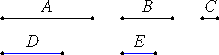# Proposition 5

Commensurable magnitudes have to one another the ratio which a number has to a number.

Let A and B be commensurable magnitudes.

I say that A has to B the ratio which a number has to a number.

Since A and B are commensurable, some magnitude C measures them.

As many times as C measures A, let so many units be in D, and, as many times as C measures B, let so many units be in E.Since C measures A according to the units in D, while the unit also measures D according to the units in it, therefore the unit measures the number D the same number of times as the magnitude C measures A. Therefore C is to A as the unit is to D. Therefore, inversely, A is to C as D is to the unit.

Again, since C measures B according to the units in E, while the unit also measures E according to the units in it, therefore the unit measures E the same number of times as C measures B. Therefore C is to B as the unit is to E.

V.22

But it was also proved that A is to C as D is to the unit, therefore, ex aequali, A is to B as the number D is to E.

Therefore the commensurable magnitudes A and B have to one another the ratio which the number D has to the number E.

Therefore, commensurable magnitudes have to one another the ratio which a number has to a number.

Q.E.D.

## Guide

This proposition equates ratios of magnitudes to ratios of numbers. Both magnitudes and numbers are represented as lines. To help distinguish them in this edition of the Elements, lines that represent numbers are drawn in blue.

The proposition states that if A = mC and B = nC, then A : B = m : n.

The proof here assumes that numbers are magnitudes, that is to say, the two definitions of proportion V.Def.5 and VII.Def.20 are compatible.

This proposition is used in X.8, its contrapositive, and a few propositions after that.

The next proposition is the converse of this one, and the two following that are its contrapositive and the contrapositive of this one. It is not clear why these four statements are separated into four propositions, but the following four statements are bundled together into one proposition X.9 instead of being separate. Perhaps originally each group of four was bundled together, but later the first group was separated.